These problems are designed for students in Grades 3-4:## Gr. 3-4: Number Sense & Numerical Operations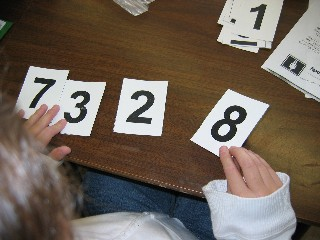•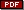Animal Shelter requires students to use fractions to figure out how many cats and dogs were available for adoption at the animal shelter.
•Tadpoles and Frogs requires students to use fractions to figure out how many tadpoles and frogs there were in the tank.
• TheGood Weather problem uses positive and negative integers in a weather setting.
• TheHigh Number Game problem requires students to compare fractions, decimals and percents.
•Ants Marching assesses student understanding of the concept of multiplication as arrays.
•Third Grade Parade also assesses student understanding of the concept of multiplication as arrays.
•Cheerleader Competition was designed to assess student understanding of multiplication as an array.
•Field Trip requires students to solve a real-life division problem and decide how to handle the remainder.
•Big Number-1 requires students to arrange the digits to form the largest 4-digit number possible and explain how they know they have formed the largest number.
•Big Number-2 requires students to arrange the digits to form the largest 4-digit number possible and explain how they know they have formed the largest number.
•Largest 3-digit Sum challenges students to arrange given digits to form the largest sum and explain their reasoning.
•Smallest 3-digit Difference challenges students to arrange given digits to form the smallest difference and explain their reasoning.
•High-Number Toss - 1 is designed to measure student understanding of place value as it is used in the Everyday Math Game of the same name.   Students must be familiar with the game to successfully solve the problem.
•High-Number Toss - 2 is designed to measure student understanding of place value as it is used in the Everyday Math Game of the same name.   Students must be familiar with the game to successfully solve the problem.
•Name That Number - 1 is designed to measure student understanding of place value as it is used in the Everyday Math Game of the same name.   Students must be familiar with the game to successfully solve the problem.
•Name That Number - 2 is designed to measure student understanding of place value as it is used in the Everyday Math Game of the same name.   Students must be familiar with the game to successfully solve the problem.
•Pattern Block Fraction Design requires students to fill a shape with pattern blocks to create a design that meets certain requirements.   Students must also write a fraction that describes the part of the total design represented by each different color pattern block.
•Fraction Game simulates a Fraction War game but students must draw a representation of each fraction and explain who won, based on the drawings.

## Gr. 3-4: Geometry & Measurement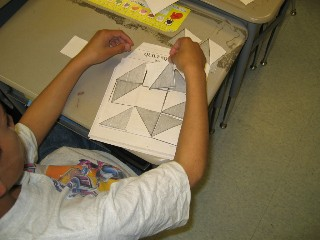• Quilt Square Challenge: Students at Flynn School in Perth Amboy, NJ, used quilt blocks and quilt mats to recreate 4x4 and 9x9 quilt block patterns.   See Quilt Square Challenge on the Quilts webpage for more information on this activity and to download the blocks and mats for students.
• InCookie Box, students must figure out which box would hold more cookies.
•Pattern Block Symmetry challenges students to use pattern blocks to fill in the shape so that the design has a vertical or horizontal line of symmetry.
•Pattern Block Symmetry: students must create a pattern block design to satisy the given conditions involving line symmetry and the number of different pattern block pieces used.
•Pattern Block Design (Grade 3) requires students to create a design to meet specified criteria.
•Pattern Block Design (Grade 4) requires students to create a design to meet specified criteria.
•Coordinate Geometry (Grade 4) requires students to plot specified points, connect the points to form a figure, then answer geometric questions about the figure.
•Area and Perimeter - 1 was designed to assess student understanding of the difference between area and perimeter.
•Area and Perimeter - 2 was designed to assess student understanding of the difference between area and perimeter.
•Shape Investigations was designed to help students develop the concept that two figures that have the same perimeter do not necessarily have the same area and vice versa.
•Pet Pens was designed to help students strengthen the concepts of perimeter and area as they use a fixed length of fencing to enclose the largest pen for a favorite pet.

## Gr. 3-4: Patterns & Algebra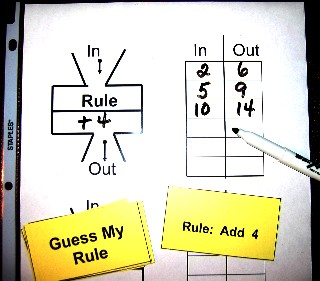•Garage Sale is a pattern problem based on dominoes.
•Favorite Shells is a pattern problem that can be solved using a picture or an input/output table.
•Fall Parade challenges students to identify the pattern formed by triangular numbers.
•Marathon Training is a pattern problem that encourages students to use an input/output table to organize the data and the solution.
•Pumpkin Picking is another pattern problem that can be solved using a picture or an input/output table. contributed by Shannon Collier, Joseph C. Caruso School, Keansburg, NJ
•Baseball Season is a pattern problem that can be easily solved using a table of values.
•Anthony's Allowance provides additional pattern practice.
•Saving Money-1 requires students to analyze the pattern in saving money to decide if the student will have enough money to buy the new CD she wants.
•Saving Money-2 requires students to analyze the pattern in saving money to decide if the student will have enough money to buy the new DVD he wants.

## Gr. 3-4: Data Analysis & Probability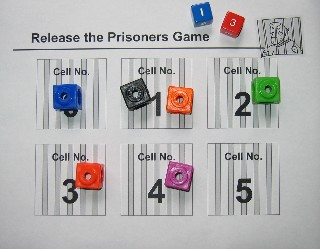•Chapter Books requires students to create a line plot, then identify the median and mode of the data.
•Carnival Tickets requires students to create a line plot, then identify the median and mode of the data.
•Lucky Draw was designed to help students develop the concept of probability. Students imagine cutting apart the letters of words and expressions, placing them in a bag, then calculating the probability of pulling different letters.
•Mathematics challenges students to calculate the probability of pulling the different letters of "MATHEMATICS" from the bag.
• TheThird Graders problem uses the letters in "THIRD GRADERS" and investigates the probability of pulling different letters from the bag.
• TheFavorite Pets problem requires students to complete a tally chart, answer questions about the data and create a bar graph of the survey results.
• IniPod Songs students must read data in a table and create a bar graph of the results.
• TheFavorite Planets survey requires students to complete the frequency chart, use the chart to answer questions about maximum and minimum, then construct a bar graph of the survey results.
•TV Survey is an example of a data analysis problem with questions designed to address each level of Bloom's Taxonomy.
•Spinner Game presents students with a frequency table of spins and requires students to create a bar graph of the results and draw a spinner that would yield those results.   Students must justify their proposed spinner, explaining how it fits the data.

## Gr. 3-4: Discrete Math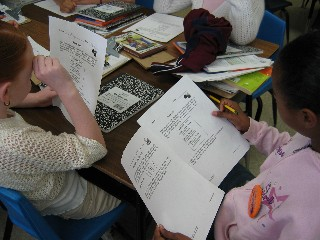•Lunch Choices investigates the combinations, given different menu choices.
• TheGame Day problem investigates the possible combinations when students are able to choose activities at Game Day centers.
•Number Draw challenges students to make all possible numbers from the three given digits.
•Number Draw Game A practices making all possible three-digit numbers, given three different digits.
•Houses in a Row challenges students to use an orderly counting system to discover how many different houses can be painted using the given colors.
•How Many Different Ways Can You Spell Math? encourages students to investigate the patterns in Pascal's Triangle to solve this problem.
•Discrete Math Combinations challenges students to figure out how many different combinations are possible for different scenarios.
•Number Draw was designed to help students explore combinations of digits used to form numbers.
•Dice Game was designed to help students explore combinations of digits to form numbers in a game setting.
• TheBoardwalk Fun problem requires students to use a logic grid to figure out what each kid wants to do at the boardwalk.   An organized clue-by-clue solution is provided for help in using this logic grid for teachers who are not familiar with these kinds of logic problems.